# Operational amplifiers

Martin McBride, 2020-05-12
Tags op-amp
Categories electronics analogue electronics analogue electronic components

An operational amplifier (or op-amp) is a high gain voltage amplifier. Op-amps are usually packaged as an integrated circuit (IC), sometimes with several op-amps in one IC.

It normally has a differential input but a single output. Here is the symbol:Since an op-amp has differential inputs, it can produce positive or negative output. This means that an op-amp typically needs three voltage rails:

• Positive +Vs
• Ground (0 volts)
• Negative -Vs

The positive and negative rails should usually be the same voltage, for example +12V and -12V is typical for modular synthesisers.

## Op-amp response to inputs

An op-amp has two input pins, a V+ and V-. It amplifies the difference between the two inputs, and the amplified voltage appears at the output.

An op-amp has a very high gain, typically tens of thousands or even hundreds of thousands. That means the even a very small voltage difference between the two input pins results in a large output voltage.

The diagram below indicates the case where the V+ input is higher than the V- input. This is a positive differential voltage, so we would expect a positive output value. Since the amplifier gain is very high, the output voltage will be very high even if the input difference is very small. In fact the output will go as high as it possibly can. It will be almost as high as the positive supply voltage +Vs. We say that the amplifier is saturated when it gets to that state - even if the input voltage goes up, the ouptut voltage can't go any higher.

For example, if the op-amp has a +/-12V supply, the saturated output might be around 10V: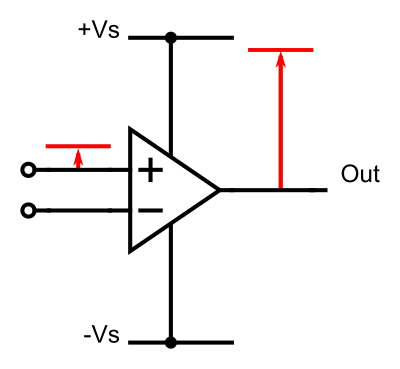The next diagram illustrates the situation when the V+ input is lower than the V- input. This is a negative differential voltage, so we will get a negative output value. Again, the amplifier will saturate, this time with a negative value. For a +/-12V supply we might get around -10V: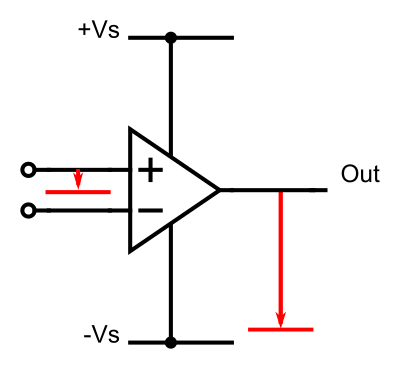The final case is when the V+ and V- inputs are equal. In that case, the differential voltage is zero, so we would expect a zero output: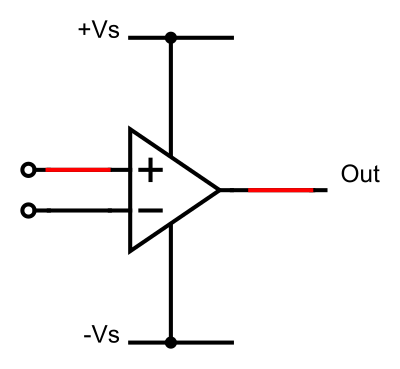However, this situation is quite unstable. Even the smallest voltage on the inputs will cause a large change in the output, so any noise on the inputs, for example, could cause wild fluctuations in the output.

## Using negative feedback

An op-amp configured like this (it is called an open loop configuration) is not hugely useful as an amplifier. We normally use negative feedback to bring things under control.

Here is a negative feedback circuit: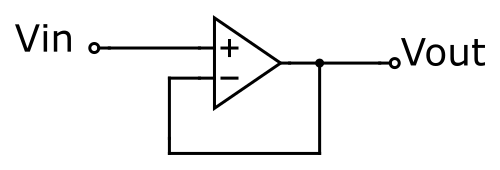Our input signal, Vin, feeds into the positive input. The output of the op-amp is fed back to the negative input.

To see how this works, imagine that we start off with Vin equal to 0V. Vin is connected to the V+ input of the op-amp. Now suppose the op-amp output is also 0V, which in turn means that the the V- input is also 0v (because it is connected to the output).

This is a state of equilibrium. V+ is 0v, V- is 0V, and since the two inputs are both equal, that means the output will be 0V.

It is also a stable equilibrium. Suppose the output voltage was slightly too high for some reason. That would cause the V- input of the op-amp to be higher than the V+ input, which is a negative differential voltage. This would cause the output voltage to reduce. In other words, any error in the output voltage will automatically correct itself, to maintain the output at 0V. The opposite is also true, if the output voltage was too low, it would create a postive differential which would drive the voltage up.

Negative feedback is much like a room thermostat. If the room is too cold it turns the heating on to warm the room. If the room is too warm it turns the heating off to let the room cool. Negative feedback corrects any error by applying a stimulus that is opposite to the error. This means that the system will always stay in the correct state.

Now suppose we increase Vin to 1V. Now the system isn't in equilibrium because there is a positive differential voltage (V+ is 1V and V- is 0V). So the output voltage increases, which increases V-. The system reaches equilibrium when the output voltage is 1V.

(Actually, equilibrium occurs when V- is very slightly below 1V, because there needs to be a small differential voltage to maintain the output at 1V. But since the gain of the op-amp is very high, the voltage difference is tiny, a fraction of a millivolt, so we tend to ignore it).

The effect of negative feedback on an op-amp is to keep V+ and V- equal. The op-amp output will take whatever value is needed to maintain this condition.

In the circuit we are looking at here, this means that the output voltage will always be the same as the input voltage. It is called a voltage follower.

We have used the example of a room thermostat, but of course an op-amp responds much faster. The rate the output voltage can change (called the slew rate) is usually around 10V per microsecond for a general purpose op-amp.

## Amplifying a signal

A voltage follower circuit can be useful in some circumstances, for example as a buffer. The input of an op-amp has very high impedance, which means it can sample a voltage while drawing almost no current, so it won't affect the circuit it is connected to.

However, it is often more useful to use an op-amp to amplify the input voltage. Here is a simple amplifier circuit: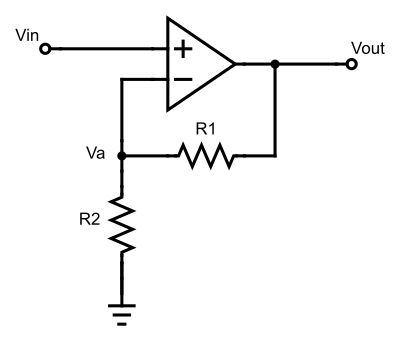The rule above still applies, negative feedback will always try to keep the two inputs equal. This means that:

Va = Vin


Now Va is connected to the output by a pair of resistors that act as a voltage divider. This means that:

Va = Vout * R2 / (R1 + R2)


Since Va is equal to Vin, this means

Vout = Vin * (R1 + R2) / R2


So the amplifier gain, Vout/Vin is:

gain = Vout/Vin = R1/R2 + 1


If we used a 100K resistor for R1, and a 10K resistor for R2, that would give a gain of 11.

## Inverting amplifier

An inverting amplifier is similar to the amplifier above, but it inverts the signal. So if the input voltage is positive, the output voltage will be negative, and vice versa. The previous circuit is often called a non-inverting amplifier.

Here is the circuit. Notice that we have flipped the op-amp symbol, so that the negative input is at the top - this just makes the circuit easier to draw.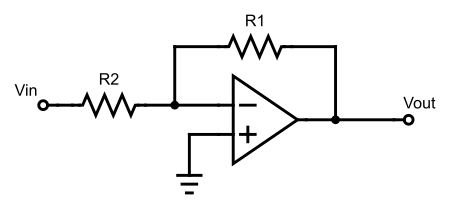In this amplifier, the positive input is tied to ground (ie 0v). The amplifier still uses negative feedback (the output is connected back to the negative input), so the same rule applies - the op-amp will try to keep the two inputs equal. In this case it means that the negative input will be kept at 0V.

Let's look at the two resistors in the circuit: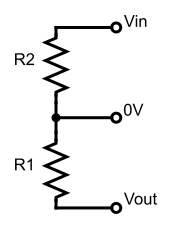Whatever input we set on Vin, the op-amp will adjust Vout so that the junction between the two resistors (which is connected to the negative input) will be zero.

The first thing to notice is that Vout must always be the opposite polarity to Vin, which is what makes this an inverting amplifier. We also know that the same amount of current is flowing through each resistor. This means (using Ohm's law):

Vout/R1 = Vin/R2


So the gain is:

gain = Vout/Vin = R1/R2


If we want an inverting voltage follower (Vout is -Vin), we just need to set the two resistors to the same value

## Inverting vs non-inverting amplifiers

There are several important differences between inverting vs non-inverting amplifiers, aside from the obvious that one inverts and the other doesn't.

Gain - A non-inverting amplifier has a gain of 1 or more. An inverting amplifier has a (negative) gain of 0 or more, in other words an inverting amplifier can make the signal smaller as well as bigger.

Input impedance - the input of a non-inverting amplifier feeds directly into the op-amp input, which has a very high impedance (it draws almost no current from the circuit it is connected to). The input of an inverting amplifier has an impedance of R2 (Vin is connected to one end of R2, and the othee end of R2 is kept at 0V, so the input current depend on R2).

Circuit complexity - the inverting amplifier is very slightly simpler in terms of the connections.

Gain calculation - the inverting amplifier has a slightly simpler gain calculation.

Certain circuits, such as a simple voltage adder, rely on the fact that the inverting input is held at 0V for correct operation.

There may be circumstances where you specifically need the signal to be inverted or non-inverted, but for audio signals this doesn't always matter. If there is no particular reason to choose one or the other, you can generally pick whichever you want.

Copyright (c) Axlesoft Ltd 2021